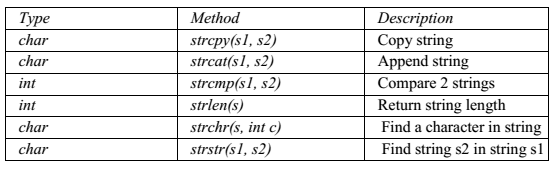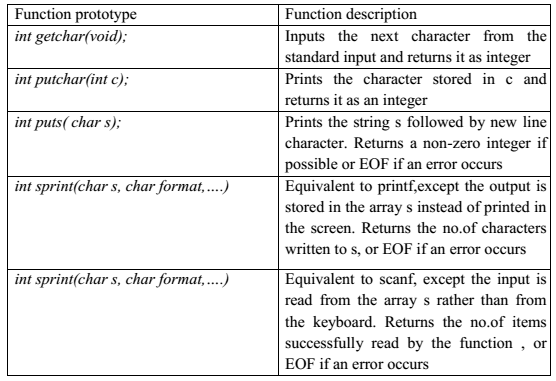# Common Functions In String A+ A  A-strcpy():
It is used to copy one string to another string. The content of the second string is copied to the content of the first string.

Syntax:
strcpy (string 1, string 2);

Example:
char mystr;

mystr = “Hello”; // Error! Illegal !!! Because we are assigning the value to mystr which is not possible in case of an string. We can only use “=” at declarations of C-String.

strcpy(mystr, “Hello”);
It sets value of mystr equal to “Hello”.
strcmp():

It is used to compare the contents of the two strings. If any mismatch occurs then it results the difference of ASCII values between the first occurrence of 2 different characters.

Syntax:
int strcmp(string 1, string 2);

```Example:
char mystr_a = “Hello”;
char mystr_b = “Goodbye”;
– mystr_a == mystr_b; // NOT allowed!
The correct way is
if (strcmp(mystr_a, mystr_b ))
printf ("Strings are NOT the same.");
else
printf( "Strings are the same.");```

Here it will check the ASCII value of H and G i.e, 72 and 71 and return the diference 1.

strcat():
It is used to concatenate i.e, combine the content of two strings.
Syntax:
strcat(string 1, string 2);

```Example:
char fname={“bob”};
char lname[]={“by”};
printf(“%s”, strcat(fname,lname));
Output:
bobby.```

strlen():
It is used to return the length of a string.
Syntax:
int strlen(string);

```Example:
char fname={“bob”};
int length=strlen(fname);
It will return 3```

strchr():
It is used to find a character in the string and returns the index of occurrence of the character for the first time in the string.

Syntax:
strchr(cstr);

```Example:
char mystr[] = "This is a simple string";
char pch = strchr(mystr,‘s’);
The output of pch is mystr```

strstr():
It is used to return the existence of one string inside another string and it results the starting index of the string.

Syntax:
strstr(cstr1, cstr2);

```Example:
Char mystr[]="This is a simple string";
char pch = strstr(mystr, “simple”);
here pch will point to mystr```

String input/output library functionsNOTE: Character arrays are known as strings.